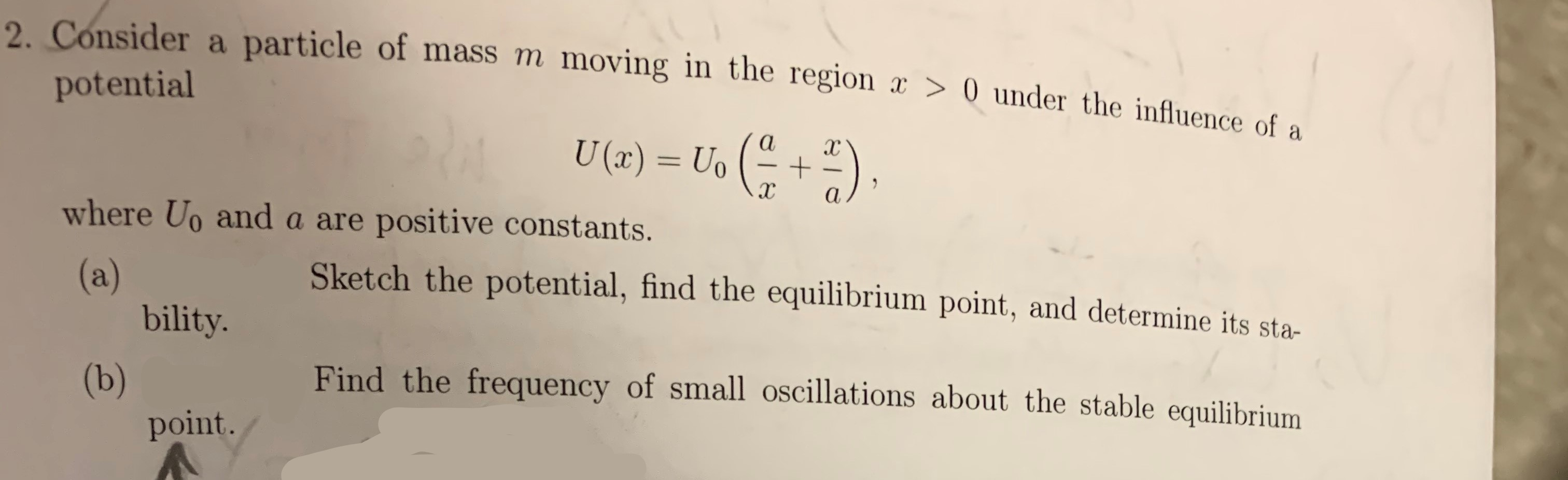# 2 Consider a particle of mass m moving in the region x > 0 under the influence of apotentialU (x) = Uoаxc+хаwhere Uo and a are positive constants.Sketch the potential, find the equilibrium point, and determine its sta-(a)bility.Find the frequency of small oscillations about the stable equilibrium(b)point.

Question
44 viewshelp_outlineImage Transcriptionclose2 Consider a particle of mass m moving in the region x > 0 under the influence of a potential U (x) = Uo а xc + х а where Uo and a are positive constants. Sketch the potential, find the equilibrium point, and determine its sta- (a) bility. Find the frequency of small oscillations about the stable equilibrium (b) point. fullscreen
check_circle

Step 1

Given,

Step 2

Part (a): Potential function can be sketched as follows,

Step 3

Equilibrium point of the functi...

### Want to see the full answer?

See Solution

#### Want to see this answer and more?

Solutions are written by subject experts who are available 24/7. Questions are typically answered within 1 hour.*

See Solution
*Response times may vary by subject and question.
Tagged in

### Physics